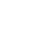How many ounces in a kilogram ? - letsdiskussBlogSatindra Chauhan

| Posted on | science-technology

# How many ounces in a kilogram ?

0
0

Ounce is the unit of measurements of mass, weight or volume. It is generally used in food packaging industry, boxing gloves manufacturing industry, fabric industry for measurement purpose. It is generally written as oz. From ounce we can convert the given quantity of material into other physical quantities such as kilogram, gram and so on. So, 1 kilogram is equal to 35. 27396195 oz. It is obtained by a certain formula which is:

m(oz)= m(kg)/ 0.02834952

where m(oz) is the mass of the substance in ounce and m(kg) is the mass in kilogram. Though there are several online convertors available for you in internet from where you can easily put the value and convert the values to required unit. But, its very important to know about the logic behind conversion and the meaning of the units used in conversion.Ounce can also be defined as 1/16th of a pound which is nearly equal to 28 grams. You will be surprised to know that ounce is the small measurement of weight.

A well-known example of measurement of ounce is the weight of a slice of bread. The name ounce is derived from Italian and Spanish word onza and that’s why it is written as oz. Ounce can also be converted to pounds and tons.

Ounces and grams both are types of units used for measurement of weight but the only difference between them is gram is used in metric system and ounce is used in United States for the measurement of weight.

You can take the help of several websites and books to understand ounce more perfectly.

0
0| Posted on

An ounce (Avoirdupois) is defined as 28.349523125 g exactly

A “kilo” is “1000” whatevers. Assuming you meant 1 kilogram:

1 kg (1000g/1kg) (1 ounce/28.349523125 g) = 35.273961949580412915675808215204 ounces0
0